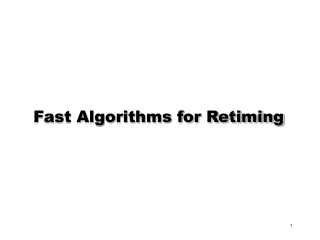DownloadDownload PresentationFast Algorithms for Retiming

# Fast Algorithms for Retiming

Télécharger la présentation## Fast Algorithms for Retiming

- - - - - - - - - - - - - - - - - - - - - - - - - - - E N D - - - - - - - - - - - - - - - - - - - - - - - - - - -
##### Presentation Transcript

1. Fast Algorithms for Retiming

2. Circuit Representation [Leiserson, Rose and Saxe (1983)] Circuit represented as retiming graph G(V,E,d,w) • V  set of gates • E  set of connections • d(v) = delay of gate/vertex v, (d(v)0) • w(e) = number of registers on edge e, (w(e)0)

3. + 7 Operation delay  3 + 7 Circuit Representation Example: Correlator (from Leiserson and Saxe) (simplified) 0 Host 0 0 0   2 3 3 0 (x, y) = 1 if x=y 0 otherwise Retiming Graph (Directed) a b Circuit Every cycle in Graph has at least one register i.e. no combinational loops.

4. 7 Preliminaries For a path p : Clock cycle Path with w(p)=0 0 0 0 0 2 3 3 0 For correlator c = 13

5. Basic Operation • Movement of registers from input to output of a gate or vice versa • Does not affect gate functionality's • Mathematical formulation: • r: V  Z, an integer vertex labeling • wr(e) = w(e) + r(v) - r(u) for edge e = (u,v) Retime by -1 Retime by 1

6. 7 7 Basic Operation Thus in the example, r(u) = -1, r(v) = -1 results in 0 0 1 0 0 1 0 0 v u u v 1 3 3 2 3 3 0 0 • For a path p: st, wr(p) = w(p) + r(t) - r(s) • Retiming: • r: VZ, an integer vertex labeling • wr(e) =w(e) + r(v) - r(u) for edge e= (u,v) • A retiming r is legal if wr(e)  0, eE

7. Retiming for Minimum Clock Cycle Problem Statement: (minimum cycle time) Given G (V, E, d, w), find a legal retiming r so that is minimized Retiming: 2 important matrices • Register weight matrix • Delay matrix

8. 7 W V0 V1 V2 V3 0 2 2 2 0 0 0 0 0 2 0 0 0 2 2 0 V0 V1 V2 V3 Retiming for minimum clock cycle W = register pathweight matrix (minimum # latches on all paths between u and v) D = path delay matrix (maximum delay on all paths between u and v) 0 0 0 v0 0 2 3 3 0 V1 V2 D V0 V1 V2 V3 V0 V1 V2 V3 0 3 6 13 13 3 6 13 10 13 3 10 7 10 13 7 c   p, if d(p)   then w(p)  1

9. Conditions for Retiming Assume that we are asked to check if a retiming exists for a clock cycle  Legal retiming: wr(e)  0 for all e. Hence wr(e) = w(e) = r(v) - r(u)  0 orr (u) - r (v)  w (e) For all paths p: u  v such that d(p)  , we require wr(p)  1 • Thus Take the least w(p) (tightest constraint) r(u)-r(v)  W(u,v)-1 Note: this is independent of the path from u to v, so we just need to apply it to u, v such that D(u,v)  

10. 7 Solving the constraints • All constraints in difference-of-2-variable form • Related to shortest path problem W V0 V1 V2 V3 D V0 V1 V2 V3 Correlator:  = 7 0 2 2 2 0 0 0 0 0 2 0 0 0 2 2 0 V0 V1 V2 V3 0 3 6 13 13 3 6 13 10 13 3 10 7 10 13 7 V0 V1 V2 V3 D>7: r(u)-r(v)W(u,v)-1 Legal: r(u)-r(v)w(e) 0 0 0 v0 0 2 3 3 0 v1 V2

11. Solving the constraints • Do shortest path on constraint graph: (O(|V||E|)) (Bellman Ford Algorithm) • A solution exists if and only if there exists no negative weighted cycle. Constraint graph D>7: r(u)-r(v)W(u,v)-1 Legal: r(u)-r(v)w(e) -1 0 -1 2 r(0) 0 r(1) 1 0 0 0 1 -1 0,-1 0 1 1 0 r(2) r(3) 0,-1 0 -1 1 A solution is r(v0) = r(v3) = 0, r(v1) = r(v2) = -1

12. Retiming To find the minimum cycle time, do a binary search among the entries of the D matrix (0(VElogV)) 7 W V0 V1 V2 V3 D V0 V1 V2 V3 0 0 0 v0 0 0 2 2 2 0 0 0 0 0 2 0 0 0 2 2 0 V0 V1 V2 V3 V0 V1 V2 V3 0 3 6 13 13 3 6 13 10 13 3 10 7 10 13 7 2 3 3 0 v1 V2 Retimed correlator: + + Retime Host Host     Clock cycle = 3+3+7=13 Clock cycle = 7 a a b b

13. Retiming: 2 more algorithms 1.Relaxation based: • Repeatedly find critical path; • retime vertex at end of path by +1 (O(VElogV)) 2. Also, Mixed Integer Linear Program formulation +1 v Critical path u

14. Retiming for Minimum Area Goal:minimize number of registers used where av is a constant.

15. Minimum Registers - Formulation Minimize: Subject to:wr(e) =w(e) + r(v) - r(u)  0 • Reducible to a flow problem

16. Problems with Retiming • Computation of equivalent initial states • do not exist necessarily • General solution requires replication of logic for initialization • Timing models • too far away from actual implementation 1 ? ? 0